# How to Compare Two Columns and Remove the Duplicate Values by Formula in Excel

To compare two columns and remove the duplicate values, we have already posted a tutorial about how to solve this problem by ‘Conditional Formatting’ feature ‘Remove Duplicate’ rule. Actually, there are some other ways to solve it as well. If you are not familiar with ‘Conditional Formatting’ feature, you can also use a formula to compare columns and remove the duplicate values. In this article, we will introduce you how to apply IF, ISERROR and MATCH functions in a formula to compare data, I hope after reading this article, you will find the way to resolve your problem.

Precondition:

See screenshot below. We prepare two lists with fruits. As the two lists are simple, so we can see ‘Apple’ exists in both two columns obviously. But if the list is very long, we cannot compare them easily, we need to use formula to compare them.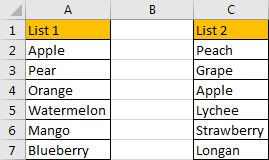## Method: Compare Two Columns and Remove Duplicates by Formula

Step 1: In B2 which is just between two columns, enter the formula =IF(ISERROR(MATCH(A2,\$C\$2:\$C\$7,0)),”Not Duplicate”,”Duplicate”).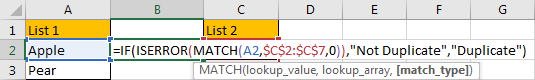Step 2: Press Enter to get value. Verify that we get ‘Duplicate’ in B2. That means ‘Apple’ in list1 also exists in list2.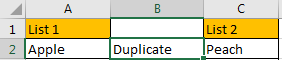Step 3: Drag the fill handle down till the end of the list.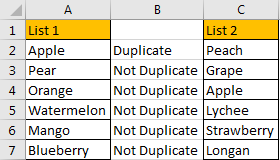Step 4: Now we already find out duplicate values, if you want to remove the duplicate value from list1, you can click B1, then click Data->Filter under Sort & Filter group.The filter dropdown list is created.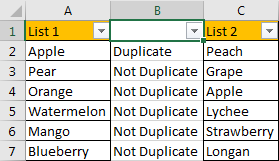Step 5: Click the small arrow button to load criteria, check on ‘Duplicate’, then click OK.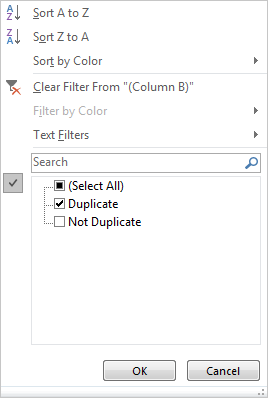Duplicate value in list1 is displayed.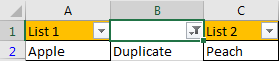Step 6: Just remove duplicate value from filtered list1. Now there is no duplicate value now.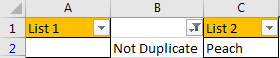### Related Functions

• Excel IF function
The Excel IF function perform a logical test to return one value if the condition is TRUE and return another value if the condition is FALSE. The IF function is a build-in function in Microsoft Excel and it is categorized as a Logical Function.The syntax of the IF function is as below:= IF (condition, [true_value], [false_value])….
• Excel ISERROR function
The Excel ISERROR function used to check for any error type that excel generates and it returns TRUE for any error type, and the ISERR function also can be checked for error values except #N/A error, it returns TRUE while the error is #N/A. The syntax of the ISERROR function is as below:= ISERROR (value)….
• Excel MATCH function
The Excel MATCH function search a value in an array and returns the position of that item.The syntax of the MATCH function is as below:= MATCH  (lookup_value, lookup_array, [match_type])….
Related Posts

Break ties with helper COUNTIF and column

Suppose you got a task to adjust the values that contain the ties; what would be your first attempt to break the ties of the given value? If you are wondering about doing this task manually, let me add that ...

Find the Closest Data to the Data Provided in Excel

In our daily work, we may encounter such an issue that to find the closest value to a certain value. In fact, Excel internal functions can help us solve this problem. In today’s article, we will show you how to ...

Abbreviate Names Or Words in Excel

As an MS Excel user, you might have come across a task where you need to abbreviate different names or words, and there are also possibilities that you might have done this task manually by assuming that there isn't any ...

Convert State Names To Abbreviations

Assume that you got a task to convert the full state's name into the abbreviations in MS Excel, and for doing this task, you might choose to do it manually, which is an acceptable way only if you don't have ...

Filter or Remove Columns

Assume that in MS Excel, you have a table consisting of a few columns consisting of few values, and you want to filter to remove the specified columns from the table. You might take it easy and would prefer to ...

Sidebar